Solving Pair of Linear Inequalities

Chapter 5 Class 11 Linear Inequalities
Serial order wise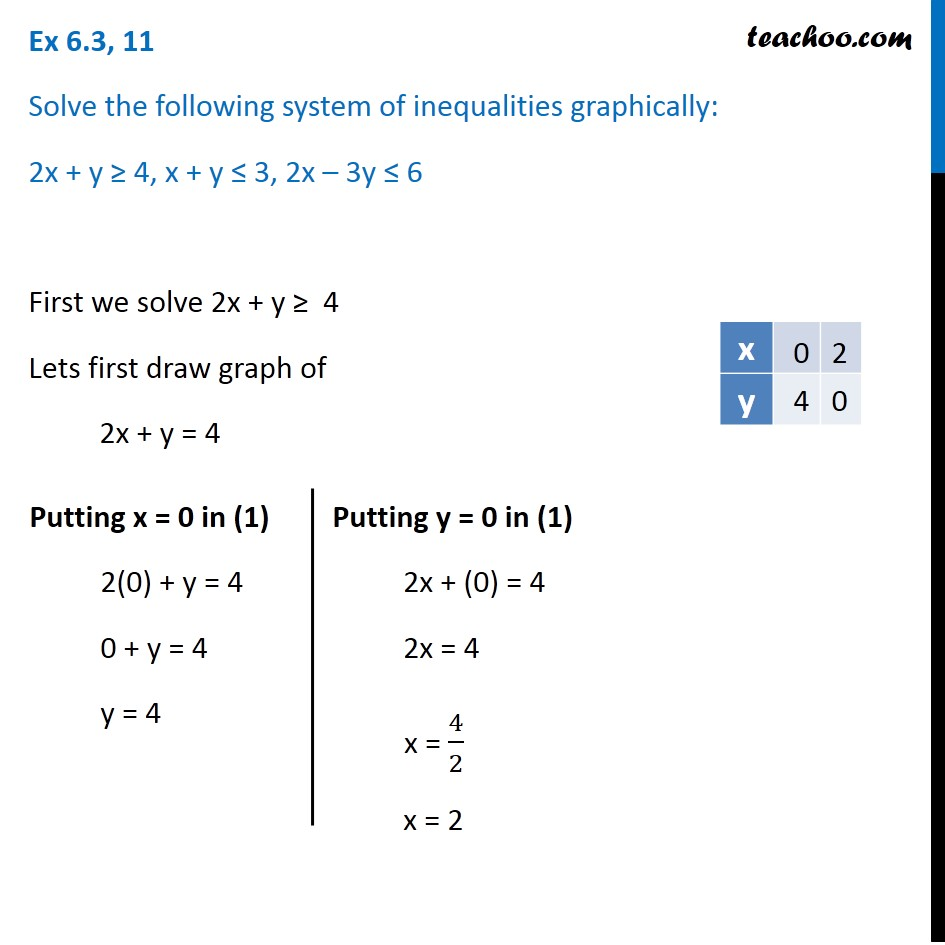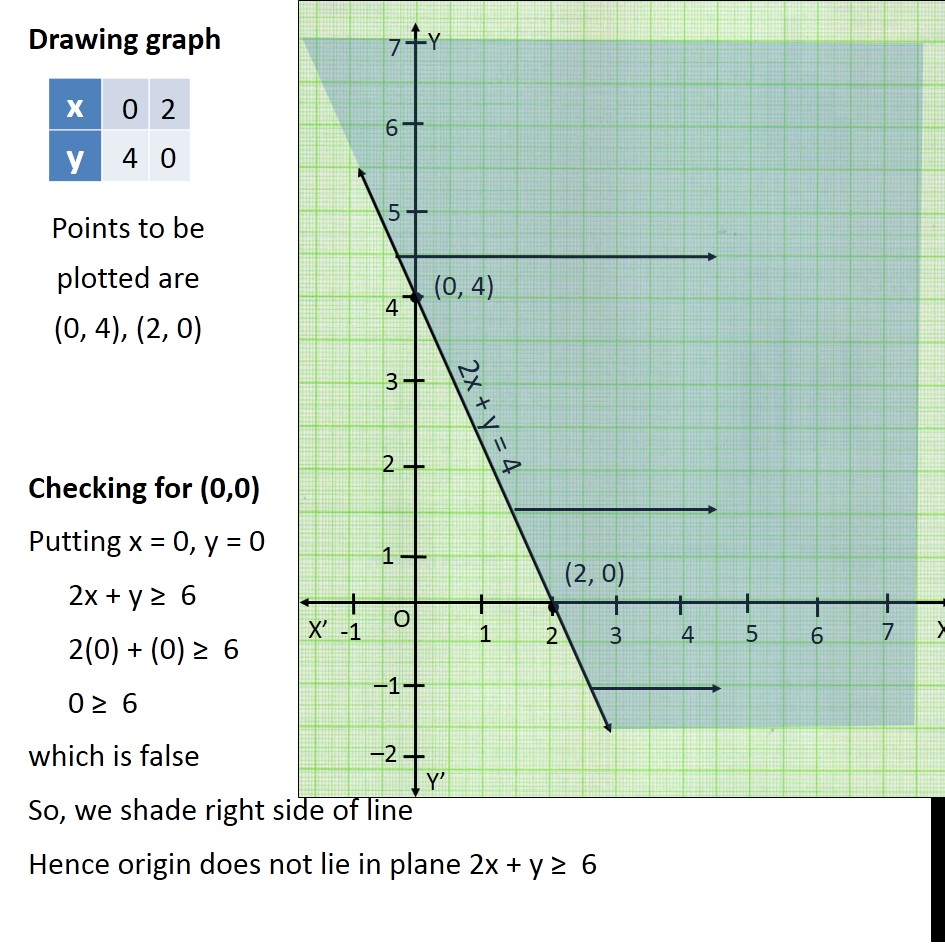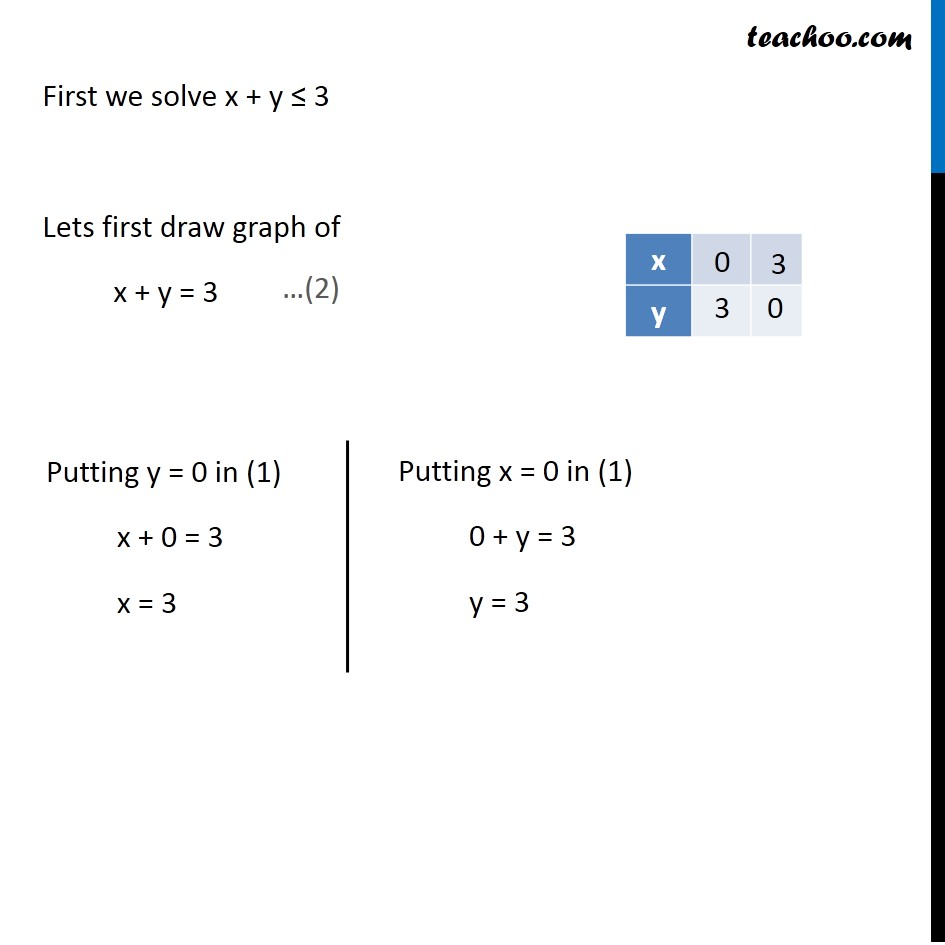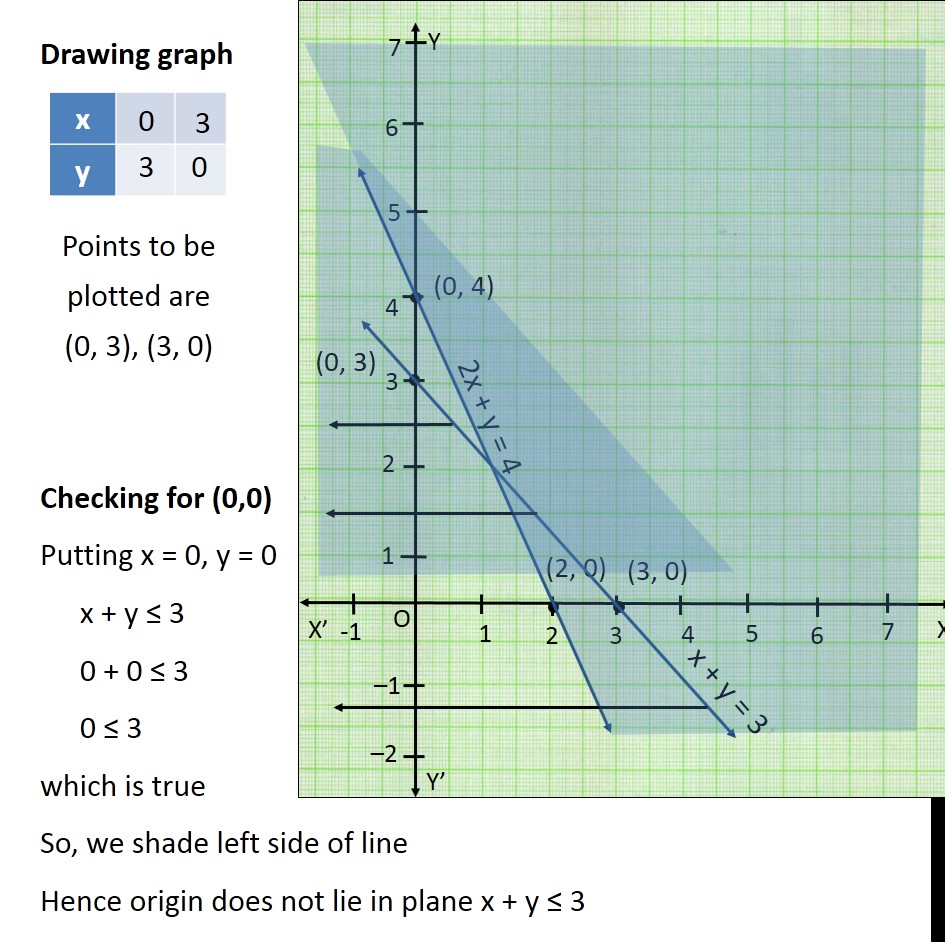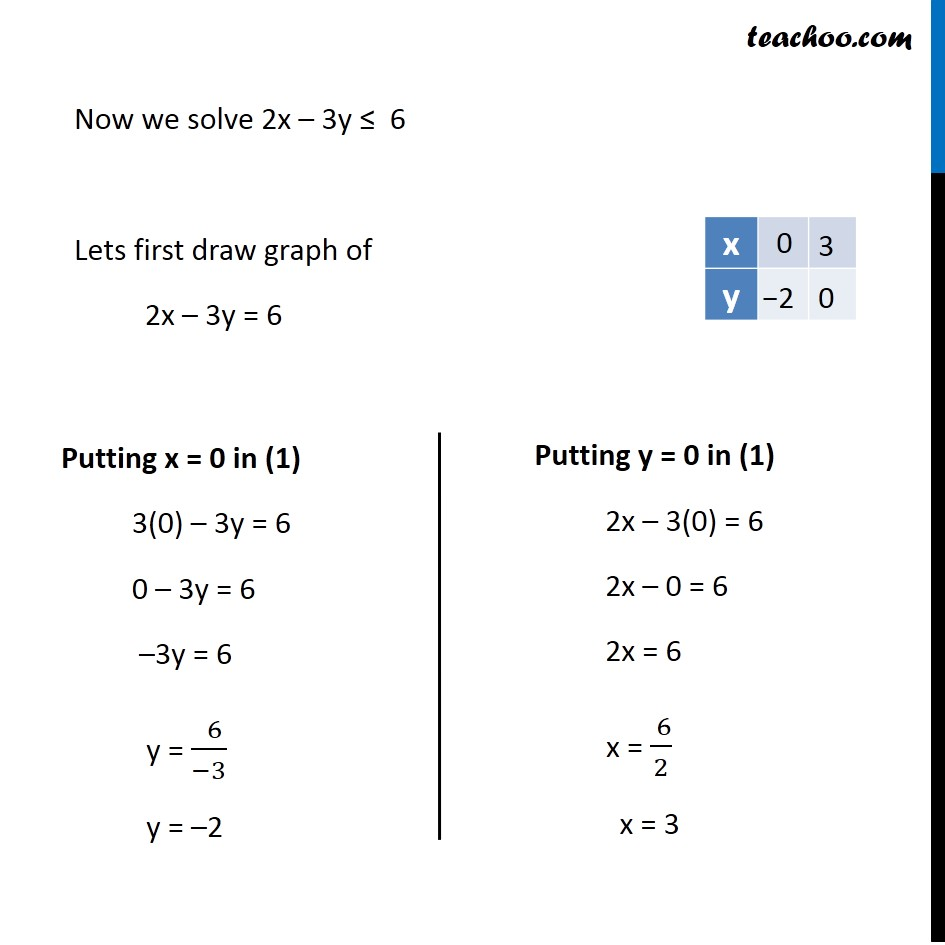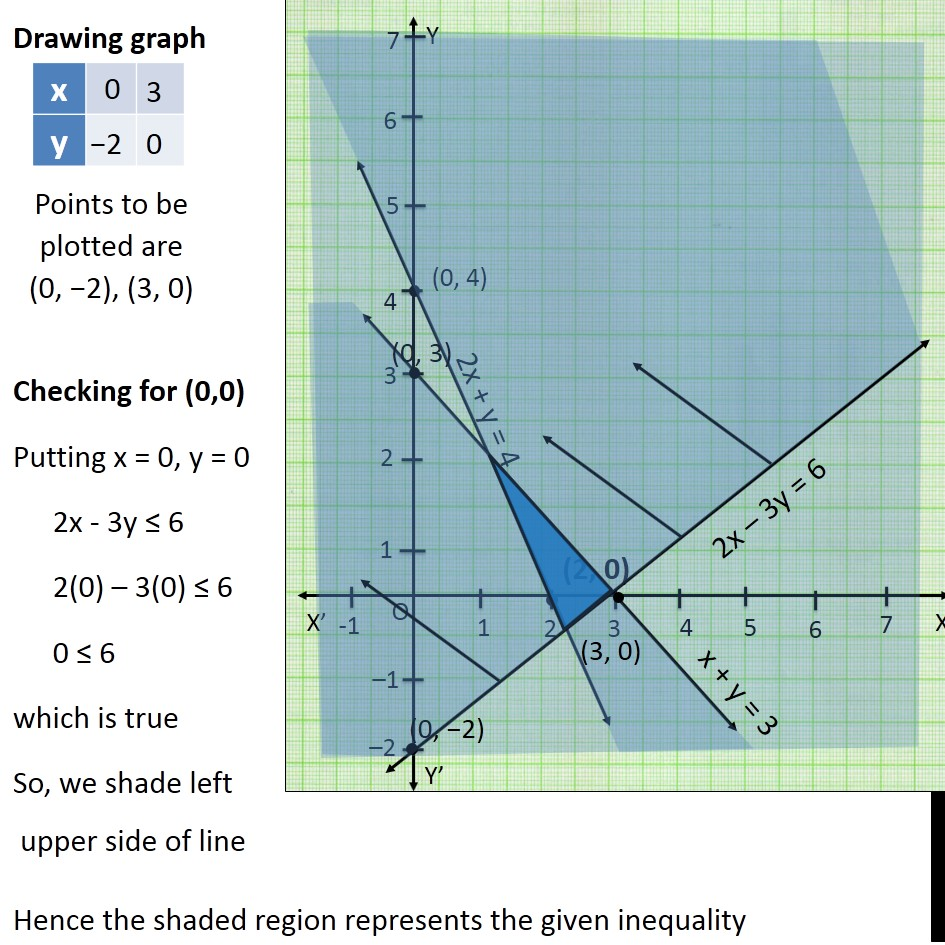Learn in your speed, with individual attention - Teachoo Maths 1-on-1 Class

### Transcript

Question 11 Solve the following system of inequalities graphically: 2x + y ≥ 4, x + y ≤ 3, 2x – 3y ≤ 6 First we solve 2x + y ≥ 4 Lets first draw graph of 2x + y = 4 Putting x = 0 in (1) 2(0) + y = 4 0 + y = 4 y = 4 Putting y = 0 in (1) 2x + (0) = 4 2x = 4 x = 4/2 x = 2 Points to be plotted are (0, 4), (2, 0) Drawing graph Checking for (0,0) Putting x = 0, y = 0 2x + y ≥ 6 2(0) + (0) ≥ 6 0 ≥ 6 which is false So, we shade right side of line Hence origin does not lie in plane 2x + y ≥ 6 First we solve x + y ≤ 3 Lets first draw graph of x + y = 3 Putting y = 0 in (1) x + 0 = 3 x = 3 Putting x = 0 in (1) 0 + y = 3 y = 3 Points to be plotted are (0, 3), (3, 0) Drawing graph Checking for (0,0) Putting x = 0, y = 0 x + y ≤ 3 0 + 0 ≤ 3 0 ≤ 3 which is true So, we shade left side of line Hence origin does not lie in plane x + y ≤ 3 Now we solve 2x – 3y ≤ 6 Lets first draw graph of 2x – 3y = 6 Putting x = 0 in (1) 3(0) – 3y = 6 0 – 3y = 6 –3y = 6 y = ( 6)/(−3) y = –2 Putting y = 0 in (1) 2x – 3(0) = 6 2x – 0 = 6 2x = 6 x = ( 6)/2 x = 3 Points to be plotted are (0, −2), (3, 0) Drawing graph Checking for (0,0) Putting x = 0, y = 0 2x - 3y ≤ 6 2(0) – 3(0) ≤ 6 0 ≤ 6 which is true So, we shade left upper side of line Hence the shaded region represents the given inequality